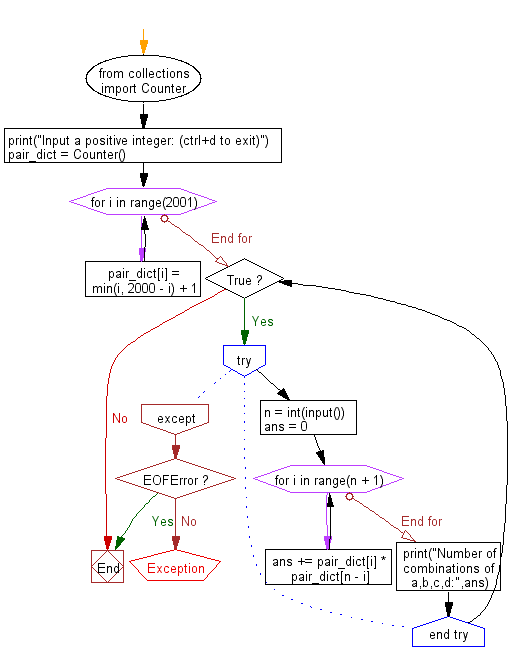﻿ Python: Find the number of combinations - w3resource# Python: Find the number of combinations

## Python Basic - 1: Exercise-62 with Solution

Write a Python program to find the number of combinations that satisfy p + q + r + s = n where n is a given number <= 4000 and p, q, r, s in the range of 0 to 1000.

Sample Solution:

Python Code:

``````from collections import Counter
print("Input a positive integer: (ctrl+d to exit)")
pair_dict = Counter()
for i in range(2001):
pair_dict[i] = min(i, 2000 - i) + 1

while True:
try:
n = int(input())
ans = 0
for i in range(n + 1):
ans += pair_dict[i] * pair_dict[n - i]
print("Number of combinations of a,b,c,d:",ans)
except EOFError:
break
``````

Sample Output:

```Input a positive integer: (ctrl+d to exit)
252
Number of combinations of a,b,c,d: 2731135
```

Flowchart:Python Code Editor:

Have another way to solve this solution? Contribute your code (and comments) through Disqus.

What is the difficulty level of this exercise?

Test your Python skills with w3resource's quiz

﻿

## Python: Tips of the Day

Python: Use Enumerate() In for Loops

```>>> students = ('John', 'Mary',  'Mike')
>>> for i, student in enumerate(students):
...     print(f'Iteration:  {i}, Student: {student}')
...
Iteration: 0, Student: John
Iteration: 1, Student: Mary
Iteration: 2, Student: Mike
>>> for i, student in enumerate(students,  35001):
...      print(f'Student Name: {student}, Student ID #: {i}')
...
Student Name: John, Student ID #: 35001
Student Name: Mary, Student ID #: 35002
Student Name: Mike, Student ID #: 35003
```